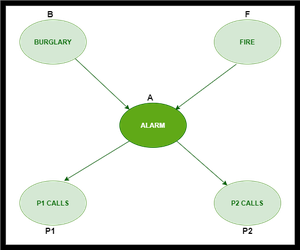# Basic Understanding of Bayesian Belief Networks

Bayesian Belief Network is a graphical representation of different probabilistic relationships among random variables in a particular set. It is a classifier with no dependency on attributes i.e it is condition independent. Due to its feature of joint probability, the probability in Bayesian Belief Network is derived, based on a condition — P(attribute/parent) i.e probability of an attribute, true over parent attribute.

(Note: A classifier assigns data in a collection to desired categories.)

• Consider this example:• In the above figure, we have an alarm ‘A’ – a node, say installed in a house of a person ‘gfg’, which rings upon two probabilities i.e burglary ‘B’ and fire ‘F’, which are – parent nodes of the alarm node. The alarm is the parent node of two probabilities P1 calls  ‘P1’ & P2 calls ‘P2’ person nodes.
• Upon the instance of burglary and fire, ‘P1’ and ‘P2’ call person ‘gfg’, respectively. But, there are few drawbacks in this case, as sometimes ‘P1’ may forget to call the person ‘gfg’, even after hearing the alarm, as he has a tendency to forget things, quick.  Similarly, ‘P2’, sometimes fails to call the person ‘gfg’, as he is only able to hear the alarm, from a certain distance.

Q) Find the probability that ‘P1’ is true (P1 has called ‘gfg’), ‘P2’ is true (P2 has called ‘gfg’) when the alarm ‘A’ rang, but no burglary ‘B’ and fire ‘F’ has occurred.

=> P ( P1, P2, A, ~B, ~F) [ where- P1, P2 & A are ‘true’ events and ‘~B’ & ‘~F’ are ‘false’ events]

[ Note: The values mentioned below are neither calculated nor computed. They have observed values ]

Burglary ‘B’ –

• P (B=T) = 0.001 (‘B’ is true i.e burglary has occurred)
• P (B=F) = 0.999  (‘B’ is false i.e burglary has not occurred)

Fire ‘F’ –

• P (F=T) = 0.002 (‘F’ is true i.e fire has occurred)
• P (F=F) = 0.998 (‘F’ is false i.e fire has not occurred)

Alarm ‘A’ –

• The alarm ‘A’ node can be ‘true’ or ‘false’ ( i.e may have rung or may not have rung). It has two parent nodes burglary ‘B’ and fire ‘F’ which can be ‘true’ or ‘false’ (i.e may have occurred or may not have occurred) depending upon different conditions.

Person ‘P1’ –

• The person ‘P1’ node can be ‘true’ or ‘false’ (i.e may have called the person ‘gfg’ or not) . It has a parent node, the alarm ‘A’, which can be ‘true’ or ‘false’ (i.e may have rung or may not have rung ,upon burglary ‘B’ or fire ‘F’).

Person ‘P2’ –

• The person ‘P2’ node can be ‘true’ or false’ (i.e may have called the person ‘gfg’ or not). It has a parent node, the alarm ‘A’, which can be ‘true’ or ‘false’ (i.e may have rung or may not have rung, upon burglary ‘B’ or fire ‘F’).

Solution: Considering the observed probabilistic scan –

With respect to the question —  P ( P1, P2, A, ~B, ~F) , we need to get the probability of ‘P1’. We find it with regard to its parent node – alarm ‘A’. To get the probability of ‘P2’, we find it with regard to its parent node — alarm ‘A’.

We find the probability of alarm ‘A’ node with regard to ‘~B’ & ‘~F’ since burglary ‘B’ and fire ‘F’ are parent nodes of alarm ‘A’.

From the observed probabilistic scan, we can deduce –

P ( P1, P2, A, ~B, ~F)

= P (P1/A) * P (P2/A) * P (A/~B~F) * P (~B) * P (~F)

= 0.95 * 0.80 * 0.001 * 0.999 * 0.998

= 0.00075

My Personal Notes arrow_drop_upCheck out this Author's contributed articles.

If you like GeeksforGeeks and would like to contribute, you can also write an article using contribute.geeksforgeeks.org or mail your article to contribute@geeksforgeeks.org. See your article appearing on the GeeksforGeeks main page and help other Geeks.

Please Improve this article if you find anything incorrect by clicking on the "Improve Article" button below.